# Calculated Members¶

Calculated members are members of a dimension or measure group that are defined based on a combination of cube data, arithmetic operators, numbers, and functions. In addition to exposing calculated members embedded in the cubes, CALUMO allows the definition of calculated members for inclusion on any view or report.

To create and use Calculated Members, use Member Explorer to associate them with a hierarchy in your cube.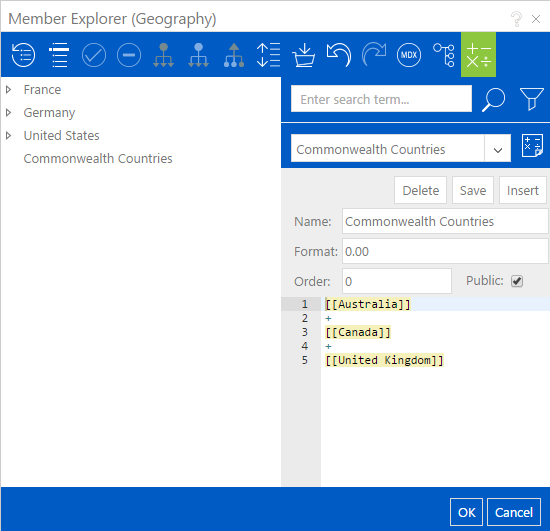## Creating a Calculated Member¶

To create a new Calculated Member, open up Member Explorer for the hierarchy that is to contain the Calculated Member in and then press the Calculated Members button on the Member Explorer toolbar.

Open Member Explorer and select the Calculated Members button on the toolbar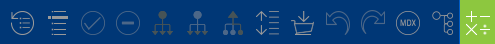On the new section that shows, press the “Create new calculated member” button.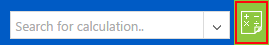This will show the Calculated Members interface as shown below with the following options available to you: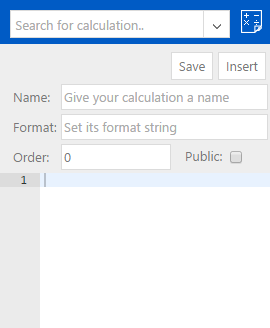### Save¶

The Save button will validate and save the Calculated Member. If the calculation is invalid, you will be notified as to why and the save will not occur.

### Insert¶

The Insert button will validate, save and then insert the Calculated Member at the bottom of the existing member tree.

### Name¶

The name of the Calculated Member, it does not need to be uniquely named, but it is recommended that you do try and name your Calculated Members as uniquely as possible for ease of use and recollection.

### Format¶

The format you want to use for the resulting numbers. This documentation from Microsoft describes acceptable formats.

### Order¶

The order in which this Calculated Member will be calculated when it is being calculated at the same time as other Calculated Members.

Info

Generally the order will not matter and you can leave this at 0.

### Public¶

If you are a CALUMO Administrator, the Calculated Member can be flagged as available to all users.

### Formula Area¶

The region in which you define the formula for your Calculated Member.

## Building The Formula¶

To build a Calculated Member formula, simply drag a member from the tree on the left to the formula area on the right. In the simple case you can use mathematical operators, or in the advanced case you can use the full power of MDX to define your formula.

The following video shows how this process works.

## Syntax and Validation¶

When a member is dragged into the formula editor, a link is established between the formula and that member so that CALUMO always knows what it represents. As you have already seen, CALUMO uses a special syntax to denote the inclusion of a member in a Calculated Members formula, [[member name]].

Within the formula editor, as shown in the screenshot below, you can see that we highlight any occurrences of these.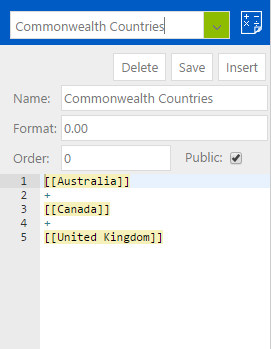With the flexibility of the formula editor, because you can put any text in there you want, there is nothing stopping a user from typing in a member in the right syntax and expecting it to work. To help stop this happening, when a Calculated Member is saved or inserted, CALUMO performs a validation on the members it knows about for the given formula against what it finds in the formula, and, if there are any discrepancies, it highlights them, notifies the user and will not save the Calculated Member. This is shown in the screenshot below: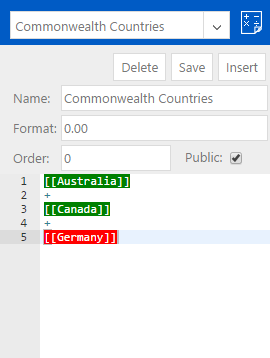To resolve this, edit the formula to remove the invalid one and drag in the correct one.